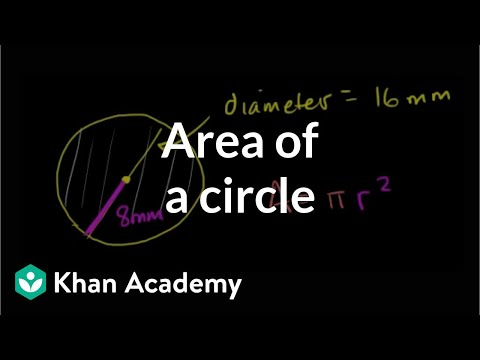Video

# Area of a shaded region (Full video)

Description: Here's a fun one: find the area of a shaded region where you first determine the area of a square and then the area of a circle. Created by Sal Khan. So the area of this would be the area of what a 10 by 10 square would be minus the area of these quarter circles. So one way to think about this is that the area of this whole red region is going to be the area of the entire square, which is 10 by 10. And then we're going to subtract out the area of the four quarter circles.

### Other videos you might be interested in### Area of a circle (Full video)